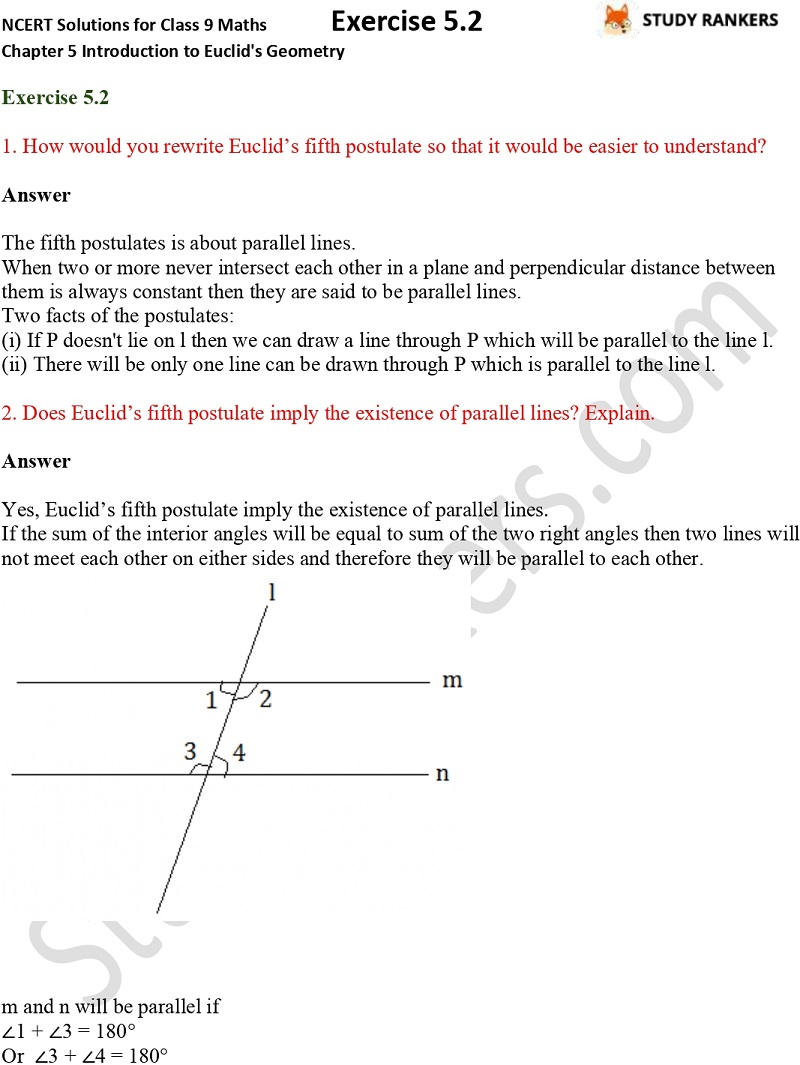## NCERT Solutions for Class 9 Maths Chapter 5 Introduction to Euclid's Geometry Exercise 5.2

NCERT Solutions of Chapter 5 Introduction to Euclid's Geometry Exercise 5.2 is provided here that will improve your problem solving skills and completing your homework. NCERT Solutions for Class 9 Maths are updated according to the latest pattern of CBSE so you can easily find your desired solutions. NCERT Solutions are the best way to explore the whole chapter and understand what the chapter is all about and important points.

Ex 5.2 contains only two questions about rewriting Euclid’s fifth postulate so that it would be easier to understand, verify if Euclid’s fifth postulate imply the existence of parallel lines.